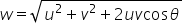science
Easy

Question

# In the parallelogram law of vectors, the cos angle denoted

## DirectionMagnitudeAngle between the vectorsNone of the aboveHint:

## The correct answer is: Angle between the vectors

### According to the parallelogram law of vector addition, if two vectors act along two adjacent sides of a parallelogram(having magnitude equal to the length of the sides) both pointing away from the common vertex, then the resultant is represented by the diagonal of the parallelogram passing through the same common vertex and in the same sense as the two vectors. Using triangle law of vector addition proves the parallelogram law of vector addition. Also, find the magnitude of the resultant vector using cosine law.Where u and v are magnitudes of the vectors. Hence cos is the angle between vectors.#### With Turito Foundation.#### Get an Expert Advice From Turito.